International
Tables for
Crystallography
Volume C
Mathematical, physical and chemical tables
Edited by E. Prince

International Tables for Crystallography (2006). Vol. C, ch. 6.4, pp. 611-612

## Section 6.4.11. Polarization

T. M. Sabinea

aANSTO, Private Mail Bag 1, Menai, NSW 2234, Australia

### 6.4.11. Polarization

| top | pdf |

The expressions for the extinction factor have been given, by default, for the σ-polarization state, in which the electric field vector of the incident radiation is perpendicular to the plane defined by the incident and diffracted beams. For this state, the polarization factor is unity. For the π-polarization state, in which the electric vector lies in the diffraction plane, the factor is. The appropriate values for the extinction factors for this state are given by multiplying F bywherever F occurs.

For neutrons, which are matter waves, the polarization factor is always unity.

For an unpolarized beam from an X-ray tube, the observed integrated intensity is given by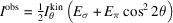. In the kinematic limit,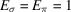, and the power to which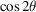is raised (the polarization index n) is 2. In the pure primary-extinction limit,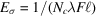, while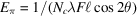. Hence, n = 1. In the pure secondary-extinction limit,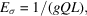where g is the mosaic-spread parameter, while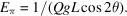Hence, n = 0. In all real cases, n will lie between 0 and 2, and its value will reflect departures from kinematic behaviour.